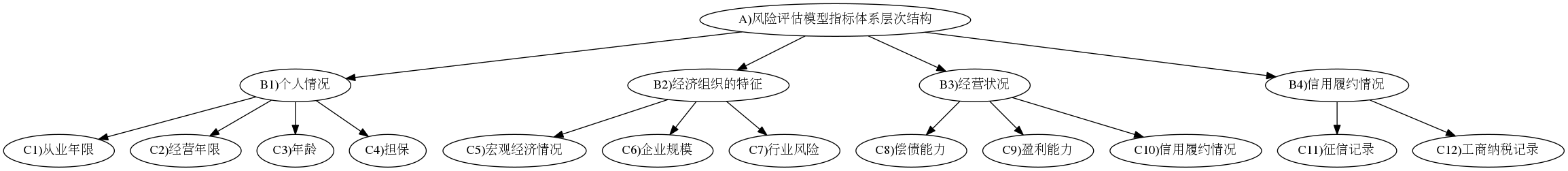@devilogic 2017-05-24T08:17:57.000000Z 字数 2141 阅读 1372

# 层次分析法

devilogic

## 层次模型## 对判断的一致性进行检验

1. 首先计算出判断矩阵中每一行运算的乘积：

1. 然后计算$M_i$$n$次方根,得到没有归一化的权重：

2. 对权重进行归一化处理，得到归一化的权重：

3. 通过归一化的权重计算出判断矩阵的最大特征根：

4. 通过判断矩阵的最大特征根计算判断矩阵的一致性指标$CR$，并通过$CR$$0.1$的比较来检验其一致性：
$CI=\frac{\lambda_{max}-n}{n-1}$,$CR=\frac{CR}{CI}$,其中，当$CR=0$时，认为判断矩阵具有完全一致性，当$CR \le 0.1$时可以认为判断矩阵一致性可以接受，各个特征之间重要性比较中不存在明显的逻辑问题。若不满足当$CR \le 0.1$，就需要对判断矩阵进行调整，直到取得满意的一致性为止。

## 利用层次分析法确定各指标的权重

1. 与业内专家访谈基础上构建一级指标判断矩阵

1. 确定权重以及一致性检验
根据公式$M_i=\sum^n_{j=1}b_{ij},(j=1,2,\ldots,n)$计算一级指标判断矩阵中的每一行元素乘积，结果如下：

然后，根据公式:$\overline{\omega}=\sqrt[n]{M_i}$计算$M_i$$n$次方根，结果如下：

接着根据公式:$\omega_i=\frac{\overline{\omega}_i}{\sum^n_{j=1}\overline{\omega}_j}$对向量进行归一化处理：

接下来对该矩阵进行一致性验证：

通过公式：$\lambda_{max}=\sum^n_{j=1}\lambda_j$计算判断矩阵的最大特征根：

上述$AW$向量每一个值是一个$\lambda_i$

B1 1 1 3 3 0.37 $\lambda_{max}=4.13$
B2 1 1 3 3 0.37 $CI=0.045$
B3 1/3 1/3 1 1/3 0.09 $CR=0.050$
B4 1/3 1/3 3 1 0.16

## 运算• 私有
• 公开
• 删除